Decimals and Fractions

Decimals and Fractions

Change a Decimal to a Fraction

To convert a decimal to a fraction, remove the decimal point from the number and place the decimal part over a denominator. That denominator should be equal to the place value of the last digit in the decimal.

Reduce the fraction to simplest form.

Example: Write 0.36 as a fraction in simplest form.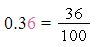6 is in the hundredths' place, so the denominator is 100.

Change a Fraction to a Decimal

To change a fraction to a decimal, divide the numerator of the fraction by the denominator.

Some decimals have repeating digits. Repeating decimals can be written with a bar over the digit(s) that repeat.

0.33333 = 0.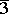One digit repeats

0.616161 = 0.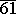Two digits repeat

Example: Change the following expression to a decimal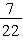First divide until the quotient ends or repeats

7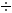22 = 0.3181818

Write a bar over the parts of the quotient which repeat

0.3181818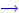0.3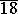Therefore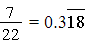Compare Fractions and Decimals

Decimals, like whole numbers and fractions, can be graphed as points on a line. In order to compare the values of fractions and decimals, a number line may be used. Numbers to the left on a number line are less than numbers to the right.

Example

Compare 0.65 to the following fraction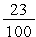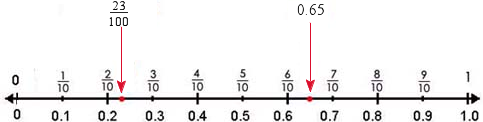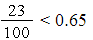It is often helpful to convert one number to another form to compare it to a given number. For example, when comparing a fraction to a decimal, you could choose to write both in decimal form.

 ones tenths hundredths 0.65 0 . 6 50 . 2 3